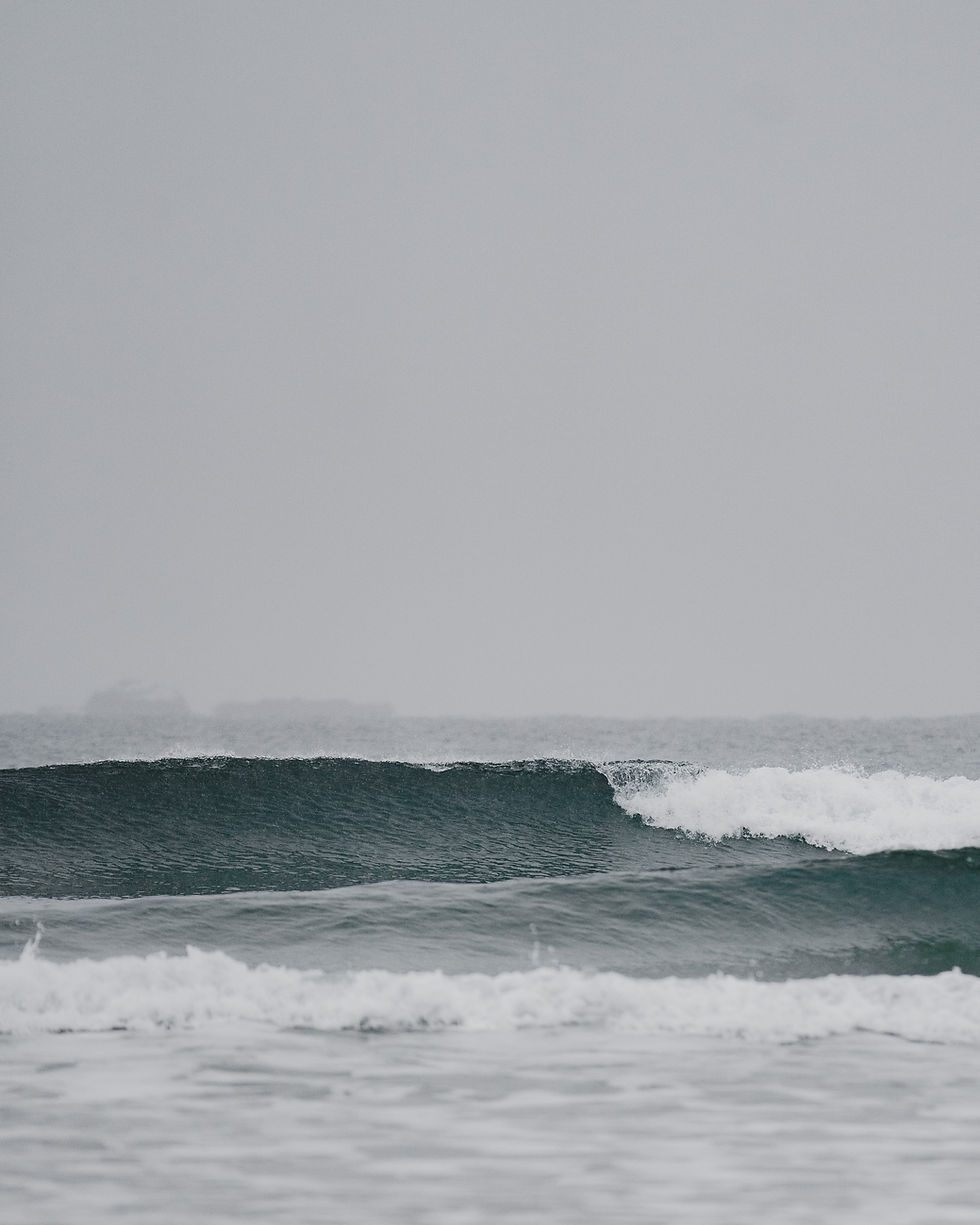1. Predicting formulae

There are many compounds that are regularly used in chemistry, so their chemical formula and name are presumed to be common knowledge. Some of them are provided in the list below:

H₂O is water,
NH₃ - ammonia,
CH₄ - methane,
CO₂ - carbon dioxide,
SiO₂ - sand.

The formulae of ionic compounds can be predicted by cross-multiplying the charges of the anion and cation and dividing them by a common factor. The name for ionic compounds is derived by combining the names of the two ions, using a suitable suffix for the anion; “ide” for single-atom ions like sulphide, “ate”/“ite” for group ions like sulphate.

When predicting the formulae of covalent molecules, the valency of each atom must be considered. The number of bonds an atom can make depends on the number of unpaired electrons present in the outer shell. Each atom in such compound make a fixed number of covalent bonds with some of them being a part of a double or a triple bond.# 1

What is the molecular formula of a covalent compound that boron and fluorine would form?

When predicting the formulae of covalent molecules, the valency of each atom must be considered. The number of bonds an atom can make depends on the number of unpaired electrons present in the outer shell.

Boron has 3 unpaired electrons in its outer shell; thus, its valency is 3. Fluorine has 1 unpaired electron in its outer shell; therefore, its valency is 1. Cross-multiplying the valencies of boron and fluorine gives BF₃. Since the subscripts have a common factor of 1, they cannot be simplified.

BF₃# 2

What is the name of LiF?

The name of ionic compounds is derived by combining the names of the two ions, using a suitable suffix for the anion; “ide” for single-atom ions like fluoride.

lithium fluoride# 3

Work out the formula of aluminium sulphate. Sulphate ion has a formula SO₄²⁻.

Aluminium is in group 3, thus its atoms each have 3 electrons in their outer shells. Since aluminium is a metal we have to add a symbol “+” to the number of valence electrons to get its most probable ionic charge 3+. Sulphate (SO₄²⁻) has a charge of 2−.

Cross-multiplying the charges of the aluminium ion and the sulphate ion gives Al₂(SO₄)₃. Since the subscripts have a common factor of 1, they cannot be simplified.

Al₂(SO₄)₃# 4

Provide the molecular formula of water.

The molecular formula of water is H₂O.

H₂O# 5

Work out the formula of calcium oxide.

Calcium is in group 2, thus its atoms each have 2 electrons in their outer shells. Since calcium is a metal we have to add a symbol “+” to the number of valence electrons to get its most probable ionic charge 2+.

Oxygen is in group 6, thus its atoms each have 6 electrons in their outer shells. Since oxygen is a nonmetal we have to subtract 8 from the number of valence electrons to get its most probable ionic charge: 6 − 8 = 2−.

Cross-multiplying the charges of the calcium ion and the oxygen ion gives Ca₂O₂. Since the subscripts have a common factor of 2, they both can be divided to give a formula of CaO.

CaOEnd of page